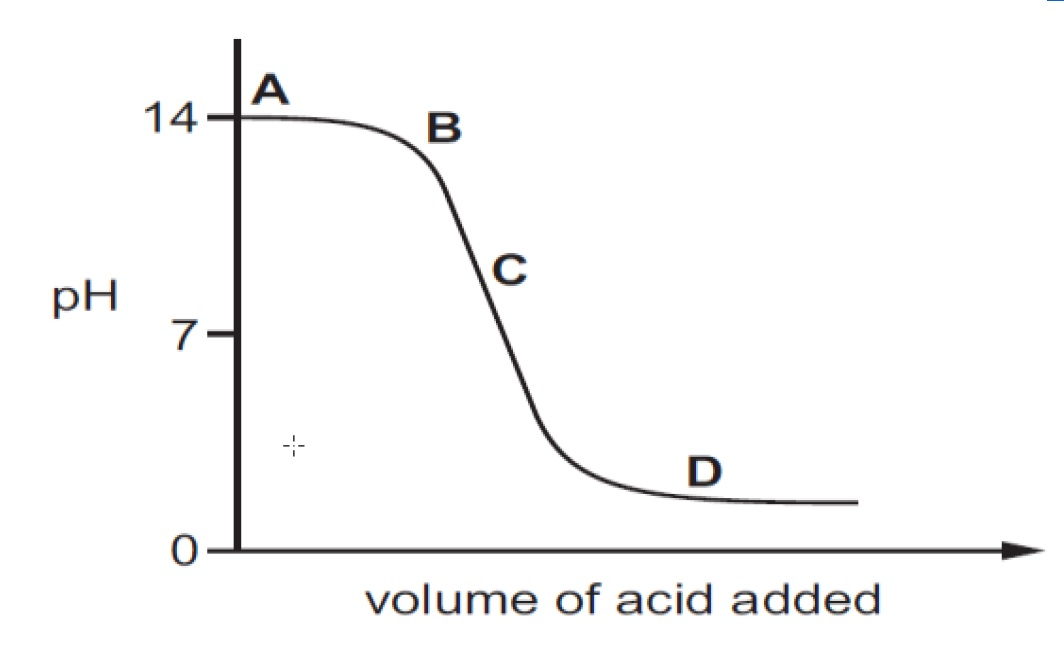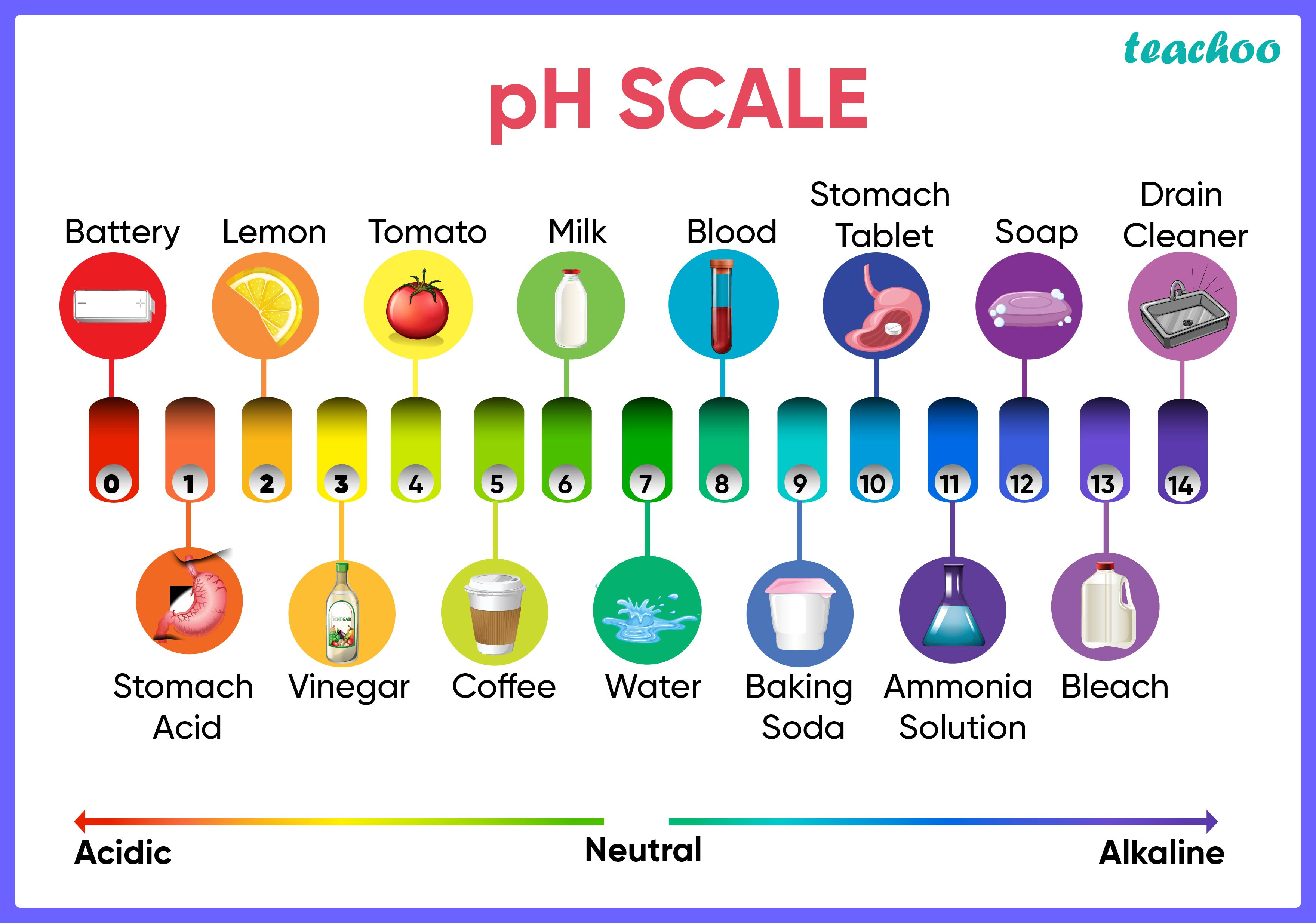Solutions - CBSE Class 10 Sample Paper for 2022 Boards - Science [MCQ]

Class 10
Solutions to CBSE Sample Paper - Science Class 10

## The graph given below depicts a neutralization reaction (acid + alkali → salt + water). The pH of a solution changes as we add excess of acid to an alkali.## (D) DWhen both acid and salt are present, the pH of the solution becomes less than 7 .

From the graph, the pH is less than 7 at only part D

So, the correct answer is (D)